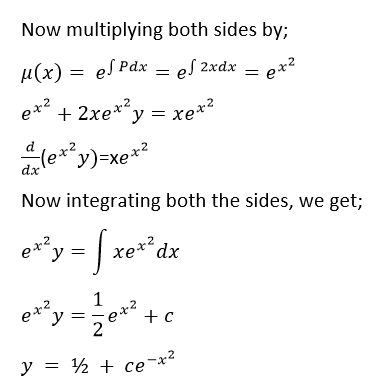# First Order Differential Equation

A first-order differential equation is defined by an equation: dy/dx =f (x,y) of two variables x and y with its function f(x,y) defined on a region in the xy-plane. It has only the first derivative dy/dx so that the equation is of the first order and no higher-order derivatives exist. The differential equation in first-order can also be written as;

y’ = f (x,y)   or

(d/dx) y = f (x,y)

The differential equation is generally used to express a relation between the function and its derivatives. In Physics and chemistry, it is used as a technique for determining the functions over its domain if we know the functions and some of the derivatives.

## First Order Linear Differential Equation

If the function f is a linear expression in y, then the first-order differential equation y’ = f (x, y) is a linear equation. That is, the equation is linear and the function f takes the form

f(x,y) = p(x)y + q(x)

Since the linear equation is y = mx+b

where p and q are continuous functions on some interval I. Differential equations that are not linear are called nonlinear equations.

Consider the first-order differential equation y’ = f (x,y),  is a linear equation and it can be written in the form

• y’ + a(x)y = f(x)

where a(x) and f(x) are continuous functions of x

The alternate method to represent the first-order linear equation in a reduced form is

(dy/dx) + P(x)y = Q (x)

Where P(x) and Q(x) are the functions of x which are the continuous functions. If P(x) or Q(x) is equal to zero, the differential equation is reduced to the variable separable form. It is easy to solve when the differential equations are in variable separable form.

## Types of First Order Differential Equations

There are basically five types of differential equations in the first order. They are:

1. Linear Differential Equations
2. Homogeneous Equations
3. Exact Equations
4. Separable Equations
5. Integrating Factor

## First Order Differential Equations Solutions

Usually, there are two methods considered to solve the linear differential equation of first order.

1. Using Integrating Factor
2. Method of variation of constant

Let us discuss each method one by one to get the solutions for differential equations of the first order.

### Integrating Factor

If a linear differential equation is written in the standard form:

y’ + a(x)y = 0

Then, the integrating factor is defined by the formula

u(x) = exp (∫a(x)dx)

Multiplying the integrating factor u(x) on the left side of the equation that converts the left side into the derivative of the product y(x)u(x).

The general solution of the differential equation is expressed as follows:

$$\begin{array}{l}y=\frac{\int u(x)f(x)dx+C}{u(x)}\end{array}$$

where C is an arbitrary constant.

### Method of Variation of a Constant

This method is similar to the integrating factor method. Finding the general solution of the homogeneous equation is the first necessary step.

y’ + a(x)y = 0

The general solution of the homogeneous equation always contains a constant of integration C. We can replace the constant C with a certain unknown function C(x). When substituting this solution into the non-homogeneous differential equation, we can be able to determine the function C(x). This approach of the algorithm is called the method of variation of a constant. However, both methods lead to the same solution.

## Properties of First-order Differential Equations

The Linear first-order differential equation possesses the following properties.

• It does not have any transcendental functions like trigonometric functions and logarithmic functions.
• The products of y and any of its derivatives are not present.

## Applications of First-order Differential Equation

Some of the applications which use the first-order differential equation are as follows:

• Newton’s law of cooling
• Growth and decay
• Orthogonal trajectories
• Electrical circuits
• Falling Body Problems
• Dilution Problems

## Problems and Solutions

Question 1 : Solve the equation y′−y−xex = 0

Solution : Given, y′−y−xe= 0

Rewrite the given equation and the equation becomes,

y′−y xex

Using the integrating factor, it becomes;

$$\begin{array}{l}u(x)=e^{\int (-1)dx}=e^{-\int dx}=e^{-x}\end{array}$$

Therefore, the general solution of the linear equation is

$$\begin{array}{l}y(x)=\frac{\int u(x)f(x)dx+C}{u(x)}=\frac{\int e^{-x}xe^{x}dx+C}{e^{-x}}\end{array}$$
$$\begin{array}{l}y(x)=\frac{\int xdx+C}{e^{-x}}=e^{x}\left ( \frac{x^{2}}{2}+C \right )\end{array}$$
.

Question 2: Solve the differential equation y’+2xy = x.

Solution: The given equation is already in a standard form, y’ + P(x)y = Q(x)

Therefore, P(x) = 2x and Q(x) = xRegister with BYJU’S – The learning app to get more information about the maths-related articles and start practice with the problems.